Home    |    Teacher    |    Parents    |    Glossary    |    About UsEstimating is an important part of mathematics and a very handy tool for everyday life. Get in the habit of estimating amounts of money, lengths of time, distances, and many other physical quantities.

Rounding off is a kind of estimating.

To round off decimals:

1. Find the place value you want (the "rounding digit") and look at the digit just to the right of it.
2. If that digit is less than 5, do not change the rounding digit but drop all digits to the right of it.
3. If that digit is greater than or equal to five, add one to the rounding digit and drop all digits to the right of it.

To round off whole numbers:

1. Find the place value you want (the "rounding digit") and look to the digit just to the right of it.
2. If that digit is less than 5, do not change the "rounding digit" but change all digits to the right of the "rounding digit" to zero.
3. If that digit is greater than or equal to 5, add one to the rounding digit and change all digits to the right of the rounding digit to zero.

Move your mouse cursor over each digit in the number below to round the number to that place.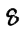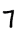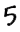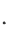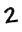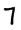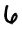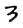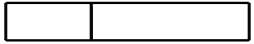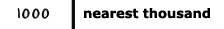Homework Help | Pre-Algebra | NumbersEmail this page to a friendSearch·  Place value·  Decimal numbers·  Estimating and     rounding·  Adding / subtracting     decimals·  Multiplying decimals·  Dividing decimals·  Percent·  Exponents·  Square roots·  Signed integers·  Adding and     subtracting integers·  Multiplying and     dividing integers·  Properties of integers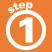First Glance In Depth Examples WorkoutEstimating and rounding decimals